HomePolymerase Chain Reaction ApplicationsOligonucleotide Quantification

# Oligonucleotide Quantification

Oligonucleotides are short, single or double-stranded polymers of nucleic acids. The concentration of nucleic acids in solution is readily quantified in a spectrophotometer when exposed to ultraviolet (UV) light at 260 nm (Figure 1). This technique works because the bases have conjugated double bonds that absorb UV light differently.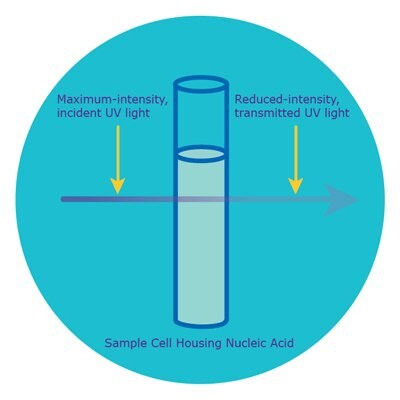Figure 1. Absorbance in a spectrophotometer.The nucleic acid in solution in the sample cell is illuminated with incident UV light at 260 nm at maximum intensity. Since the nucleic acid bases absorb some of the UV light, the transmitted UV light is reduced in intensity. Absorbance is determined by the following formula:Where: A260 = absorbance at 260 nm; Io = intensity of incident light; and, I = intensity of transmitted light.

Following an absorbance reading, the Beer-Lambert Law comes into play as it relates the amount of absorbance to the concentration of the absorbing nucleic acid as follows:Where:

A260 = absorbance at 260 nm

𝜀260 = extinction coefficient of the oligonucleotide at 260 nm in L ⋅ mol-1 ⋅ cm-1

c = concentration in mol L-1

= pathlength in cm

Key to the Beer-Lambert law is the extinction coefficient, which measures how strongly the absorbing bases reduce the intensity of the UV light from incidence to transmission. Since the absorbance of each base is different, base composition, base order, and sequence length will influence the final absorbance of the particular nucleic acid undergoing quantification. Therefore, the greatest accuracy is achieved when the extinction coefficient is calculated for each oligonucleotide. The nearest-neighbors model is ideal for oligonucleotides, and the formula is as follows: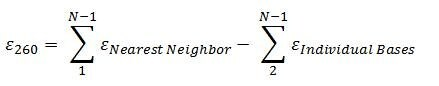Tables 1 and 2 display the nearest-neighbor and individual-bases extinction coefficients to be used in the nearest-neighbors formula when calculating the final, total extinction coefficient of an oligonucleotide.

Table 1. Nearest-neighbor extinction coefficients in L ⋅ mol-1 ⋅ cm-1The nearest-neighbor formula treats bases in the nearest-neighbor term as dinucleotides, so extinction coefficients are determined for pairs by reading from the 5' to 3' position. For example, for the tetranucleotide 5'-ACGT-3', there are three dinucleotides (AC, CG, and GT). The extinction coefficients for these pairs are 21,200, 18,000, and 20,000 L ⋅ mol-1 ⋅ cm-1, respectively.
Table 2. Individual-bases extinction coefficients in L ⋅ mol-1 ⋅ cm-1The nearest-neighbors formula treats bases in the individual-bases term individually, so extinction coefficients are one per each base.

Given that the pathlength of the sample cell is 1.0 cm, all inputs to the Beer-Lambert law are now accounted for, and so the equation can be rearranged to calculate the concentration of the oligonucleotide in question.

To calculate concentration, the Beer-Lambert law is rearranged as follows: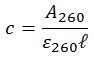## Example: Calculation for 5'-ACGT-3'

Step one: calculate the extinction coefficient

𝜀260 = (AC+CG+GT)-(C+G) L ⋅ mol-1 ⋅ cm-1

𝜀260 = (21,200+18,000+20,000)-(7,400+11,500) L ⋅ mol-1 ⋅ cm-1

𝜀260 = 40,300 L ⋅ mol-1 ⋅ cm-1

On all technical online displays and print documentation, the above value of 40,300 would be displayed as 40.3. That is because the units are L ⋅ mmol-1 ⋅ cm-1 (millimole instead of mole).

Step two: calculate the concentration

A260 is set to 1.0 optical density (OD) unit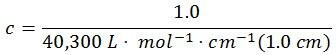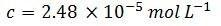Step three: calculate the molar mass (MM)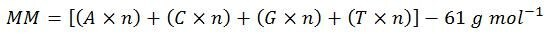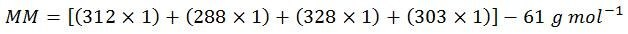Normally, ‘molecular weight’ is used in place of ‘molar mass,’ but since the units of mass of a mole are needed for these calculations, ‘molar mass’ is the most correct term. Also, for simplification of display, the above molar masses of each base have been rounded to the nearest whole number. Therefore, the calculated value of 1,170 is slightly below the true value of 1,173.83.

Step four: convert the concentration to customary units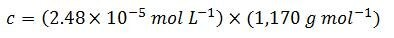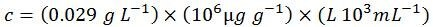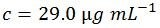Therefore, for this particular sequence: 5'-ACGT-3', 1 OD = 29.0 µg mL-1 (one OD is the amount of oligonucleotide in 1 mL of solution that exhibits an A260 of 1.0 in a light pathlength of 1.0 cm). As a comparison, here is the value as determined by OligoEvaluator™, our online oligonucleotide sequence calculator:There is excellent agreement between the manually calculated value of 29.0 and the OligoEvaluator calculated value of 29.1 (the discrepancy is because rounded molar masses of each base were used in the manual calculation as explained above in step three).

In addition to OligoEvaluator, 29.1 is the value that would be displayed on the technical datasheet provided with this oligonucleotide.

## Shortcut Calculation for 5'-ACGT-3'

The above longform calculation steps are tedious. The following formula combines all steps into one and therefore can be used as a shortcut:## Modifications

The only difference between the calculation of concentration for a standard and modified oligonucleotide is that the molar mass of the modification is taken into account and added to the total molar mass of the oligonucleotide. For example, if our above sequence:

● 5'-ACGT-3'

is changed to

● 5'-6-FAM™-ACGT-3'

and, the new molar mass of 1,711.3 g mol-1 is plugged into either the longform calculations or shortcut formula, the new concentration is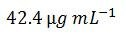This value agrees well with the value determined by OligoEvaluator: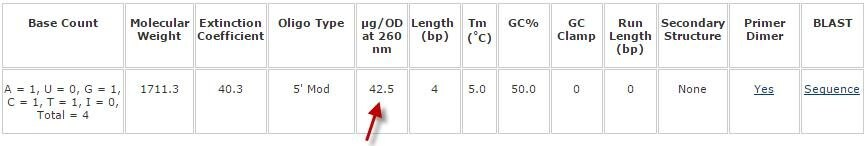## Quick Conversions

As a rule of thumb, experiments have validated the following quick conversions (ds = double stranded; ss = single stranded):

● A260 dsDNA = 50 µg mL-1
● A260 ssDNA = 37 µg mL-1
● A260 ssRNA = 40 µg mL-1

Without performing any calculations, these conversion factors provide reasonable estimates for longer sequences with more even distribution of A, C, G, and T bases, such as those created by the shearing of genomic DNA (for the dsDNA estimate). However, with oligonucleotides, the base composition is more likely to be skewed, and therefore, these estimates are unreliable (often overestimate the concentration). The concentration must be determined for each oligonucleotide as outlined above using either the longform or shortcut calculation. Either the longform or shortcut calculation can be used to verify quantification values provided with technical datasheets.